International
Tables for
Crystallography
Volume F
Crystallography of biological macromolecules
Edited by M. G. Rossmann and E. Arnold

International Tables for Crystallography (2006). Vol. F, ch. 19.5, pp. 447-449   | 1 | 2 |

Section 19.5.7. Determination of structures

R. Chandrasekarana* and G. Stubbsb

aWhistler Center for Carbohydrate Research, Purdue University, West Lafayette, IN 47907, USA, and  bDepartment of Molecular Biology, Vanderbilt University, Nashville, TN 37235, USA
Correspondence e-mail:  chandra@purdue.edu

19.5.7. Determination of structures

| top | pdf |

If the amplitude and phase of each diffracted wave are known, structure determination is, in principle, straightforward (Section 19.5.3.4). In practice, however, the phase problem for fibres is more acute than for single crystals because of the limited resolution of the data, and because the diffracted intensities overlap as a result of disorientation and cylindrical averaging. Patterson methods (MacGillavry & Bruins, 1948; Stubbs, 1987) have sometimes been useful, but the cylindrically averaged Patterson function is usually too complicated for detailed interpretation. Phasing by heavy-atom methods is not practical for polymers with small unit cells because of the difficulties in incorporating heavy atoms into the structures. Structures having small unit cells are instead determined by constructing initial models based on chemical information and the observed helical parameters. Extensions of the isomorphous-replacement method (Namba & Stubbs, 1985) have been useful in determining structures, such as those of helical viruses, in which the unit cells are much larger. In all cases, refinement and evaluation of the model structures are essential. A flow chart of the sequential steps in the determination and refinement of fibre structures with small unit cells is shown in Fig. 19.5.7.1.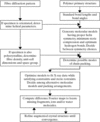Figure 19.5.7.1 | top | pdf |Flow chart of the principal steps in the determination and refinement of fibre structures with small unit cells.

19.5.7.1. Initial models: small unit cells

| top | pdf |

For many biopolymers, especially polypeptides, polynucleotides and polysaccharides, the repeating unit is a monomer or a small oligomer and the unit-cell dimensions are in the range 10 to 50 Å. Such unit cells can accommodate one or more polymer helices, packed in an organized fashion.

An initial model is constructed from the primary structure of the repeating unit, using bond lengths, bond angles and some conformation angles derived from surveys of accurate single-crystal analyses. The model must satisfy the observed helical parameters and have reasonable intra- and inter-chain non-bonded, hydrogen-bonded and polar interactions.

This preliminary model provides an approximate solution to the phase problem and a starting point for refinement. Since there is no assurance that the refined model represents the true structure, however, stereochemically plausible alternatives must be carefully considered, refined and objectively adjudicated. Alternatives can include both right- and left-handed helices, single helices, and multistranded helices with parallel and antiparallel strands. The next stage involves the packing arrangement in the unit cell. If two or more helices are present, their positions, orientations and relative polarities must be varied in refinement.

19.5.7.2. Refinement: small unit cells

| top | pdf |

The widely used linked-atom least-squares (LALS) technique (Arnott & Wonacott, 1966; Smith & Arnott, 1978) and the variable virtual bond (PS79) method (Zugenmaier & Sarko, 1980) were developed for fibre structures. They are similar in principle to the least-squares refinement procedure for crystalline proteins (Hendrickson, 1985), although bond lengths and bond angles are usually kept fixed in the fibre refinements. The function minimized by the LALS program is of the form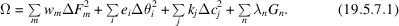The first term on the right-hand side is the weighted sum of the squares of the differences,, between observed and calculated X-ray structure amplitudes of Bragg reflections or continuous diffraction. Either or both types of data can be used as necessary. The weights,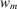, are inversely proportional to the estimated variance of the data. The second term minimizes the differences,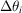, between the expected (standard) values of conformation and bond angles and those in the model; the weights,, are based on empirically determined variances. The third term is designed to take care of non-bonded interactions and thus keep the model free from steric compression. It includes the deviations from target values of both intra- and inter-chain hydrogen bonds and the differences between acceptable and calculated non-bonded distances for those contacts that are smaller than the acceptable limiting values. The weights,, are based on the Buckingham energy function for non-bonded contacts and empirical variances for hydrogen bonds. Finally, the fourth term imposes constraints (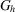, with Lagrange multipliers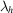) for helix connectivity and ring closure, as in a furanose or pyranose, and it vanishes when all such constraints are satisfied. During the refinement, the structure factors are calculated with either the conventional atomic scattering factor f or with a solvent-corrected atomic scattering factor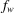(Fraser et al., 1978; Chandrasekaran & Radha, 1992) given by the function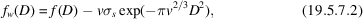where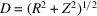,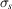is the electron density of the solvent and v is the excluded volume of the atom. If the van der Waals radius of water is taken as 2 Å,for water is 0.2984 e Å−3. Equation (19.5.7.2) allows for the solvent contribution to the diffracted intensity and is particularly useful in studying hydrated fibres in which structured and amorphous water can account for up to 50% of the total mass.

19.5.7.3. Data-to-parameter ratio

| top | pdf |

The total number of data used in this refinement process is M + I + J, where M, I and J are, respectively, the number of observations in the first three terms of equation (19.5.7.1). If P is the number of parameters refined and H is the number of independent constraints in the last term, then the number of degrees of freedom of the system is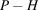. The effective number of data is given by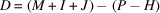. The data-to-parameter ratio (D/P), a measure of the dependability of the final results, must be greater than one for meaningful refinement. D/P is typically in the range 3 to 11 in the analysis of polynucleotide and polysaccharide structures. This ratio is comparable to those commonly reported for single-crystal structures, confirming that fibre-diffraction analysis of polymers, despite the limited number of X-ray data, can yield reliable results.

19.5.7.4. Initial models: large unit cells

| top | pdf |

For large macromolecular aggregates, such as viruses and cytoskeletal filaments, initial models cannot usually be devised using the primary structure of the molecule alone. The largely α-helical filamentous bacteriophages form a rare class of exceptions (Makowski et al., 1980). Molecular-replacement methods, in which initial models are constructed from single-crystal structure determinations of the separated components of the aggregate or from known related structures, can be useful, but because of the limited number of data in a fibre pattern such models can sometimes be difficult to refine.

Multi-dimensional isomorphous replacement (MDIR), an extension of the isomorphous-replacement method of protein crystallography, has been useful in studying helical viruses (Stubbs & Diamond, 1975; Namba & Stubbs, 1985). The dimensions are the real and imaginary parts of the various overlapping structure factors at a given point in the diffraction pattern. Information about both the phases of the structure factors and the relative magnitudes of the overlapping structure factors is obtained from heavy-atom derivatives of the virus; at least twice as many heavy-atom derivatives as the number of significant G terms in equation (19.5.3.7) are required. If the structure of a related aggregate is known, MDIR can be combined with molecular replacement (Namba & Stubbs, 1987a; Wang & Stubbs, 1994); in this case, fewer derivatives are required.

Layer-line splitting (Franklin & Klug, 1955) arises when the helical symmetry of the scattering particles is close to, but not exactly, integral. For example, tobacco mosaic virus (TMV) has 49.02 subunits in three turns of the viral helix. In this case, the G terms in each layer line do not fall at exactly the same Z values in the diffraction pattern. The resulting shifts in the positions of the layer lines can be measured for the native aggregate and, in favourable cases, for heavy-atom derivatives, and used to provide additional phase information (Stubbs & Makowski, 1982). Information from electron microscopy (Beese et al., 1987) and neutron scattering (Nambudripad et al., 1991) has also been used.

19.5.7.5. Refinement: large unit cells

| top | pdf |

Refinement of fibre structures having large unit cells has many parallels to refinement in protein crystallography. Refinement in real space, especially the solvent-flattening approach, has been widely used to improve electron-density maps and is particularly valuable in structure determination of noncrystalline fibres. Since helical aggregates have finite radii, g terms [equation (19.5.3.6)] can be set to zero outside a maximum radius and back-transformed to obtain refined estimates of the phases of the G terms. More detailed solvent-flattening algorithms can also be used (Namba & Stubbs, 1985).

Molecular models can be refined by methods conceptually related to those of LALS. The principal difference is that bond lengths and angles are not kept fixed, but are restrained to remain close to standard values. The restrained least-squares method (Hendrickson, 1985), widely used in protein crystallography, has been adapted (Stubbs et al., 1986) for fibre diffraction and used to refine a number of filamentous virus structures (Namba et al., 1989; Nambudripad et al., 1991). Although effective, the radius of convergence of this method is less than desired, probably because of the limited number of data available from fibre diffraction (Wang & Stubbs, 1993).

Molecular-dynamics methods have been used to increase the radius of convergence of refinement (Wang & Stubbs, 1993). The program X-PLOR (Brünger et al., 1987) has been adapted for fibre diffraction and can handle data from both crystalline and noncrystalline fibres. A potential-energy function of the form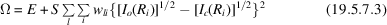is minimized. The first term, E, is an empirical energy function that accounts for distortions in bond lengths, bond angles and conformation angles, and for non-bonded, electrostatic and hydrogen-bonding interactions. The second term accounts for the differences between the observed and calculated X-ray intensities at specific values ofon every layer line l;is the weight for each observation and S is a normalizing factor. In the most effective use of this method, simulated annealing, the process of heating the structure to a temperature of 3000 to 4000 K is simulated, then the structure is cooled (`annealed') in small increments. At high temperatures, energy barriers between the starting model and structures of lower potential can be overcome; in this way, the radius of convergence of the refinement is increased.

19.5.7.6. Difference Fourier methods

| top | pdf |

As in crystallography, difference maps are used during refinement to correct errors and to identify missing fragments of the model and, in the final stages of refinement, to identify solvent molecules and associated ions.

In crystalline fibre diffraction, the most common difference maps use calculated phases with amplitudes of eitheror. In both cases, weighting the coefficients on the basis of the observed and calculated structure amplitudes has been used to minimize the root-mean-square error in the electron-density maps. Reflections superposed by cylindrical averaging do, however, present problems. One solution is to divide the observed intensity equally among the superposed reflections. This is a reasonable approach in the initial stages of structure analysis, when the reliability of the model is uncertain, and has the advantage of minimizing bias toward the model. Alternatively, the observed intensity may be split in the same ratio as the calculated intensity. This approach, although biased, is more effective for locating solvent molecules and ions in an otherwise well determined structure. Difference Fourier maps have played a significant role in determining the molecular structures and packing arrangements in unit cells mediated by water molecules and cations of several polynucleotide (Chandrasekaran et al., 1995, 1997) and polysaccharide helices (Winter et al., 1975; Chandrasekaran et al., 1988, 1998; Chandrasekaran, Radha & Lee, 1994).

In noncrystalline fibre diffraction, the superposition of intensities due to cylindrical averaging is more serious and must be taken into account. Namba & Stubbs (1987b) have shown that the coefficients yielding the most accurate electron-density maps of the full structure have amplitudes of, where N is the number of significant terms in equation (19.5.3.7) (the number of superposed intensities), and the observed intensity is divided in the ratio of the calculated intensity. For filamentous viruses at moderate resolution, N is typically in the range four to six. As in crystallography and crystalline fibre diffraction, maps calculated from amplitudes ofhave low noise levels and are most useful for checking the accuracy of final models and for locating solvent molecules.

19.5.7.7. Evaluation

| top | pdf |

As in crystallography, fibre structures are evaluated by statistical measures, such as R values, and by the examination of difference maps. Fibre-diffraction R values are inherently lower than those expected in crystallography, particularly when large numbers of intensities have been superposed by cylindrical averaging (Stubbs, 1989). The largest likely R value for noncrystalline TMV at 3 Å resolution is about 0.31 and for polycrystalline DNA at 3 Å resolution it is about 0.41, both significantly less than the value of 0.59 to be expected from noncentric single-crystal analyses (Millane, 1989).

Comparison of R values alone is not necessarily a reliable way to discriminate between competing models. Such discrimination is often required for structures with small unit cells, for which alternative models are routinely refined (Sections 19.5.7.1and 19.5.7.2). The relative merits of any pair of competing models can be assessed on the basis of several types of statistics (Arnott, 1980) using Hamilton's significance test (Hamilton, 1965), which considers not only residuals but also numbers of degrees of freedom (Section 19.5.7.3). Such a test is essential. There are many examples in the literature where R values have been lowered by the simple process of increasing the number of degrees of freedom; a decreased R value obtained in this way may or may not have any significance.

Difference Fourier maps have been used to evaluate crystalline fibre diffraction analyses for many years, for example, to reject the controversial Hoogsteen base pairing in double-stranded DNA (Arnott et al., 1965), and later to discriminate between 10- and 11-fold double helices of RNA (Arnott et al., 1967). Difference maps have been essential in the refinement of fibre structures with large unit cells (Namba et al., 1989; Wang & Stubbs, 1994), both to identify errors in early models and to confirm that the final structures contained no major errors or omissions.

References

Arnott, S. (1980). Twenty years hard labor as a fiber diffractionist. Am. Chem. Soc. Symp. Ser. 141, 1–30.Google Scholar
Arnott, S., Wilkins, M. H. F., Fuller, W. & Langridge, R. (1967). Molecular and crystal structures of double-helical RNA. III. An 11-fold molecular model and comparison of the agreement between the observed and calculated three-dimensional diffraction data for 10- and 11-fold models. J. Mol. Biol. 27, 535–548.Google Scholar
Arnott, S., Wilkins, M. H. F., Hamilton, L. D. & Langridge, R. (1965). Fourier synthesis studies of lithium DNA. Part III: Hoogsteen models. J. Mol. Biol. 11, 391–402.Google Scholar
Arnott, S. & Wonacott, A. J. (1966). The refinement of the crystal and molecular structures of polymers using X-ray data and stereochemical constraints. Polymer, 7, 157–166.Google Scholar
Beese, L., Stubbs, G. & Cohen, C. (1987). Microtubule structure at 18 Å resolution. J. Mol. Biol. 194, 257–264.Google Scholar
Brünger, A. T., Kuriyan, J. & Karplus, M. (1987). Crystallographic R-factor refinement by molecular dynamics. Science, 235, 458–460.Google Scholar
Chandrasekaran, R., Bian, W. & Okuyama, K. (1998). Three-dimensional structure of guaran. Carbohydr. Res. 312, 219–224.Google Scholar
Chandrasekaran, R., Puigjaner, L. C., Joyce, K. L. & Arnott, S. (1988). Cation interactions in gellan: an X-ray study of the potassium salt. Carbohydr. Res. 181, 23–40.Google Scholar
Chandrasekaran, R. & Radha, A. (1992). Structure of poly d(A)·poly d(T). J. Biomol. Struct. Dynam. 10, 153–168.Google Scholar
Chandrasekaran, R., Radha, A. & Lee, E. J. (1994). Structural roles of calcium ions and side chains in welan: an X-ray study. Carbohydr. Res. 252, 183–207.Google Scholar
Chandrasekaran, R., Radha, A. & Park, H.-S. (1995). Sodium ions and water molecules in the structure of poly d(A)·poly d(T). Acta Cryst. D51, 1025–1035.Google Scholar
Chandrasekaran, R., Radha, A. & Park, H.-S. (1997). Structure of poly d(AI)·poly d(CT) in two different packing arrangements. J. Biomol. Struct. Dynam. 15, 285–305.Google Scholar
Franklin, R. E. & Klug, A. (1955). The splitting of layer lines in X-ray fibre diagrams of helical structures: application to tobacco mosaic virus. Acta Cryst. 8, 777–780.Google Scholar
Fraser, R. D. B., MacRae, T. P. & Suzuki, E. (1978). An improved method for calculating the contribution of solvent to the X-ray diffraction pattern of biological molecules. J. Appl. Cryst. 11, 693–694.Google Scholar
Hamilton, W. C. (1965). Significance tests on the crystallographic R factor. Acta Cryst. 18, 502–510.Google Scholar
Hendrickson, W. A. (1985). Stereochemically restrained refinement of macromolecular structures. Methods Enzymol. 115, 252–270.Google Scholar
MacGillavry, C. H. & Bruins, E. M. (1948). On the Patterson transforms of fibre diagrams. Acta Cryst. 1, 156–158.Google Scholar
Makowski, L., Caspar, D. L. D. & Marvin, D. A. (1980). Filamentous bacteriophage Pf1 structure determined at 7 Å resolution by refinement of models for the α-helical subunit. J. Mol. Biol. 140, 149–181.Google Scholar
Millane, R. P. (1989). R factors in X-ray fiber diffraction. II. Largest likely R factors. Acta Cryst. A45, 573–576.Google Scholar
Namba, K., Pattanayak, R. & Stubbs, G. (1989). Visualization of protein–nucleic acid interactions in a virus: refinement of intact tobacco mosaic virus at 2.9 Å resolution by fiber diffraction data. J. Mol. Biol. 208, 307–325.Google Scholar
Namba, K. & Stubbs, G. (1985). Solving the phase problem in fiber diffraction. Application to tobacco mosaic virus at 3.6 Å resolution. Acta Cryst. A41, 252–262.Google Scholar
Namba, K. & Stubbs, G. (1987a). Isomorphous replacement in fiber diffraction using limited numbers of heavy-atom derivatives. Acta Cryst. A43, 64–69.Google Scholar
Namba, K. & Stubbs, G. (1987b). Difference Fourier syntheses in fiber diffraction. Acta Cryst. A43, 533–539.Google Scholar
Nambudripad, R., Stark, W. & Makowski, L. (1991). Neutron diffraction studies of the structure of filamentous bacteriophage Pf1 – demonstration that the coat protein consists of a pair of α-helices with an intervening, non-helical loop. J. Mol. Biol. 220, 359–379.Google Scholar
Smith, P. J. C. & Arnott, S. (1978). LALS: a linked-atom least-squares reciprocal-space refinement system incorporating stereochemical restraints to supplement sparse diffraction data. Acta Cryst. A34, 3–11.Google Scholar
Stubbs, G. (1987). The Patterson function in fiber diffraction. In Patterson and Pattersons, edited by J. P. Glusker, E. K. Patterson & M. Rossi, pp. 548–557. New York: Oxford University Press.Google Scholar
Stubbs, G. (1989). The probability distributions of X-ray intensities in fiber diffraction: largest likely values for fiber diffraction R factors. Acta Cryst. A45, 254–258. Google Scholar
Stubbs, G. & Makowski, L. (1982). Coordinated use of isomorphous replacement and layer-line splitting in the phasing of fiber diffraction data. Acta Cryst. A38, 417–425.Google Scholar
Stubbs, G., Namba, K. & Makowski, L. (1986). Application of restrained least-squares refinement to fiber diffraction from macromolecular assemblies. Biophys. J. 49, 58–60.Google Scholar
Stubbs, G. J. & Diamond, R. (1975). The phase problem for cylindrically averaged diffraction patterns. Solution by isomorphous replacement and application to tobacco mosaic virus. Acta Cryst. A31, 709–718.Google Scholar
Wang, H. & Stubbs, G. (1993). Molecular dynamics in refinement against fiber diffraction data. Acta Cryst. A49, 504–513.Google Scholar
Wang, H. & Stubbs, G. (1994). Structure determination of cucumber green mottle mosaic virus by X-ray fiber diffraction. Significance for the evolution of tobamoviruses. J. Mol. Biol. 239, 371–384.Google Scholar
Winter, W. T., Smith, P. J. C. & Arnott, S. (1975). Hyaluronic acid: structure of a fully extended 3-fold helical sodium salt and comparison with the less extended 4-fold helical forms. J. Mol. Biol. 99, 219–235.Google Scholar
Zugenmaier, P. & Sarko, A. (1980). The variable virtual bond modeling technique for solving polymer crystal structures. Am. Chem. Soc. Symp. Ser. 141, 225–237.Google Scholar